## Vibrational Motion - Complete Toolkit

### Objectives

1. To identify several examples of vibrating objects and to use terms such as equilibrium position, restoring force, fixed end, and damping to describe their motion.
2. To describe an object undergoing periodic motion using terms such as sinusoidal, cycle, period, amplitude, and damping and to relate these terms to the position-time graph.
3. To define period, frequency, and amplitude and to determine their values from verbal and graphical descriptions of a vibrating object's motion.
4. To describe and explain the motion of a simple pendulum using such representations as a free-body diagrams, position-time graphs, velocity-time graphs, energy tables, equations for period, and terms such as position, velocity, and acceleration.
5. To describe and explain the motion of a vibrating mass on a spring using such representations as a free-body diagrams, position-time graphs, velocity-time graphs, energy tables, energy bar charts, equations for period, and terms such as position, velocity, and acceleration.

### Interactive Simulations

1.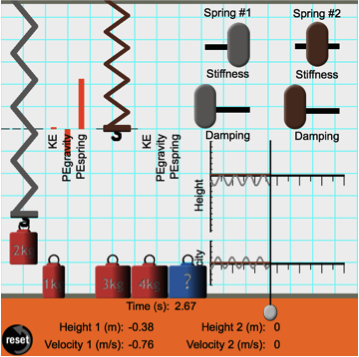Physics Interactive: Mass on a Spring

This simulation from The Physics Classroom's Physics Interactives section animates the vibrational motion of a mass on a spring. The position-time and velocity-time graph of the motion are represented in real-time. Bar charts showing the kinetic energy, gravitational potential energy, and elastic potential energy are also shown in real-time. Users can alter the mass that is hung on the spring, the stiffness of the spring, and the amount of damping. Two springs are included for conducting side-by-side comparative studies. This variable-rich environment allows students to explore a variety of relationships related to vibrating masses on springs. The Physics Classroom has prepred a ready-to-use exercise that focuses on the question of what variables affect the period and the frequency of the vibrating mass.

2.PhET:  Hooke’s Law Simulation

As 17th-century physicist Robert Hook determined, “As the extension, so the force.” This newer HTML5 simulation lets students stretch and compress springs to explore the relationships among force, spring constant, displacement, and potential energy. It will help them gain insight into the meaning of “restoring force” an area of documented student misconception. It also promotes understanding of the predictable mathematical relationships that underlie Hooke’s Law. Teachers: The simulation can be set up as a spring system in either series or parallel. In addition, click the “Energy” tab to explore how potential energy stored in the spring changes with spring constant (k) and displacement.

3.PhET:  Pendulum Lab

This simulation displays one or two pendulums to explore how the period of a simple pendulum depends on the length of the string, mass of the pendulum bob, and amplitude of the swing. Use the Photogate timer to easily measure the period! You can vary the friction or jump to Planet X to explore the effect of changing gravity on a pendulum. Teachers: You will want to check out the PhET “Gold Star” teacher-submitted activities for great lesson plans to accompany this simulation.

4.Hooke’s Law Digital Lab

This two-hour digital lab for high school physics was created specifically to accompany the PhET simulation “Masses and Springs”. In the first lesson, students explore how displacement of a spring is mathematically related to the load applied to it. In the next day’s exploration, learners analyze the energy of a mass oscillating on a spring by observing distribution and transfer of kinetic, potential, and gravitational potential energy. Turn-Key Resource: includes explicit directions for use of the simulation, problem sets, rubric, and answer key.

### Video and Animations

1.Direct Measurement Videos: SHM with Motion Graphs

Once again, veteran HS physics teacher Peter Bohacek has created a great DVM (Direct Measurement Video) to support conceptual understanding of the kinematics of simple harmonic motion (SHM). This video shows a low-friction glider moving on an air track. Springs on each side apply forces to the glider that produce SHM. Graphs of position, velocity, and acceleration vs. time are simultaneously displayed.

2.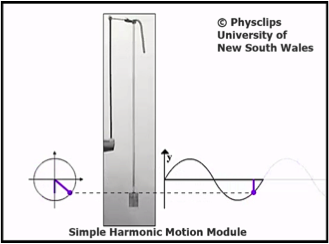PhysClips:  Simple Harmonic Motion

This animation-based tutorial was recently rewritten to HTML. It would be a good choice for students with disabilities or reading difficulties – it presents information in a non-textual way, but without sacrificing rigor. The animations are nicely constructed, blending video and diagrams, with narration by a physics instructor with a lively Australian accent! This module takes the learner through a 4-part learning cycle: 1) Overview of simple harmonic motion, 2) Angular velocity, amplitude, and phase, 3) displacement, velocity, and acceleration in SHM, and 4) Acceleration and vibration. You’ll also find links to content support for teachers, chladni patterns, and phasor addition.

3.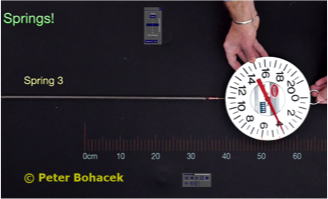Direct Measurement Video: Spring Force

Here you’ll find seven high-resolution videos that allow students to use digital rulers and frame-counters to analyze applied force on springs and make precise measurements of quantities such as position and time. This set of videos, produced by HS teacher Peter Bohacek, allows students to measure the force and elongation of seven steel springs. Their data can be used to find the spring force constant of each spring. The resource can also be used to demonstrate Hooke’s Law.

4.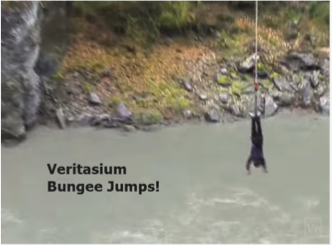Veritasium: When Is a Bungee Jumper’s Acceleration Max?

At what point in a bungee jump is acceleration the greatest? Physics education researcher and YouTube icon Derek Muller brings us another cool “think problem” that lets you integrate concepts of kinematics, gravitational acceleration, spring tension, and the restoring force. The 1-minute video offers students 5 choices – Acceleration is greatest at A) Immediately after leaping off the platform, B) When the bungee cord becomes taut, C) At the fastest point, D) At the very bottom of the jump, or E) On the rebound. Teachers: Most students erroneously pick “C”, confusing velocity with acceleration. Each choice links to a short video explaining why it was correct or incorrect.  Link to full video of Dr. Muller’s jump:  Full Bungee Jump Video

5.Circus Physics:  Pendulum Motion

This video-based resource examines factors that affect the amplitude and period of a pendulum. It provides a highly visual way to explore pendulum motion as a trapeze artist swings on a bar/rope system. Watch what happens to the pendulum period as her center of mass changes when she sits on the bar or moves to the rope below. The accompanying activity guide introduces the math associated with pendulum motion. (Includes a Teacher’s Guide with discussion questions and classroom activities.)

### Labs and Investigations

1. The Physics Classroom, The Laboratory, A Wiggle in Time

Students observe the motion of a mass on a spring using a motion detector and describe the motion with words, graphs, and numbers.

2. The Physics Classroom, The Laboratory, Period of a Pendulum

Students investigate the variables that affect the period of a pendulum and determine a mathematical equation that relates period to the variables that affect it.

### Problem-Based Learning Activity: Real-World Applications

1.Problem-Based Learning: Bungee Jumping

This activity for introductory physics presents a rough design for a bungee jump from a 20-meter tower. Students will work cooperatively to figure out the parameters for a safe jump. While some information is given, learners are expected to research certain aspects, such as the medically-recommended maximum acceleration for an untrained jumper. In keeping with the PBL method, students sift through information to separate useful from irrelevant data, locate missing information on their own, and the apply physics in finding solutions (including determining the spring constant of the bungee cord). Note to Teachers: The Student Guide is freely accessible. Access to the Teacher’s Guide with answer key is available by contacting the authors.

### Interactive Digital Homework Problems

1.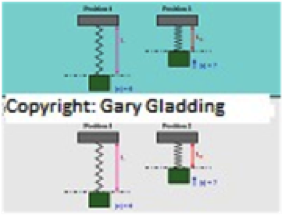Mass on a Vertical Spring Problem

If you haven’t yet seen Gary Gladding’s Interactive Examples, get ready to be impressed. Each problem consists of a conceptual exploration, strategic analysis that encourages critical thinking, and explicit help with setting up the calculations. In this interactive problem, a mass hangs from a vertical spring. If the spring is stretched and then released, what is the speed of the block when it returns to its original position for the first time? Students will use the Conservation of Mechanical Energy method to solve the problem. The author anticipates conceptual roadblocks and helps students recognize when and how to use energy conservation principles to solve physics problems.

2.Block and Spring SHM Problem

This resource presents a similar, but not identical system to the previous problem. A block is attached to a massless spring on a friction-free surface. Students are given the initial velocity and distance from equilibrium. At what time will the block next pass through the x = 0 point?  This problem was designed to help learners make the connection between the oscillation of a mass on a spring and the sinusoidal nature of simple harmonic motion. It provides carefully sequenced “help” support that includes free-body diagram, Displacement vs. Time graphs depicting SHM, and explicit help in using the Work-Kinetic Energy Theorem to solve the problem.

### Classroom Learning Module: Understanding Periodic Motion

1. TeachEngineering: Into the Swing of Things

After watching a 1940 clip of the Galloping Gertie bridge collapse and a teacher demo with a simple pendulum, student groups do Internet research to identify examples of harmonic or periodic motion. Their task is to answer guided questions about factors that affect pendulum motion; basic properties of periodic motion; and how engineers exploit periodic motion to gain a mechanical advantage. This lesson includes background information, content support for teachers, vocabulary definitions, and both pre-lesson and post-lesson assessment ideas.

2.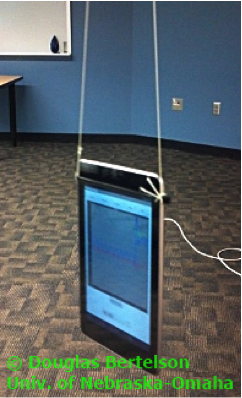TeachEngineering: Android Pendulums

Here you’ll find the hands-on activity that accompanies the first lesson. Students will use Android smartphones or tablets as the bobs of a simple pendulum system that hangs from the ceiling. Why Android devices? Because they can be loaded with the AccelDataCapture app that was developed by the author of this module. The lesson explains how to alter one variable while keeping all other parameters constant. Students will identify the independent/dependent variables, collect data, and use the simple pendulum equation. Detailed set-up instructions, data sheets, and assessments with answer key are provided.

### Problem-Solving Exercises:

1. The Calculator Pad, Wave Basics, Problems #1-4

### Science Reasoning Activities:

1. The Period of a Pendulum

2. Mass on a Spring

### Historical Context:

1.The Story Behind the Science:  Pendulum Motion

Bet you’ve never heard of this wonderful collection of 30 science stories developed to help students explore key science concepts through the eyes of the real people who were involved. This “Story” explores the humble pendulum and its significant role in the development of modern science. Inspired by Galileo’s classic experimentation, the pendulum provided the Western world’s first accurate means of timekeeping. But perhaps more importantly, the story of the pendulum brings to light the shift to the use of mathematics to understand the natural world – the methodological core of the Scientific Revolution. It also delves into the value of idealization in science.

### Common Misconception:

1. Confusion of Amplitude and Period

A vibrating object tends to lose energy over the course of time. This is referred to as damping. Damping causes the amplitude of vibration to decrease from one cycle to the next cycle. Students observing this will often use the phrase "slowing down" to describe the decrease in amplitude. They may even suggest that the period is decreasing. Such statements need to be corrected for a variety of reasons. First, the vibrating object is constant increasing and decreasing its speed from cycle to cycle. The increases in speed occur as the object is approaching its equilibrium position and these increases defy any description of the object "slowing down." Second, the tendency to confuse a decrease in amplitude with a decrease in period demonstrates a huge confusion on the student's part. The period of vibration remains constant from cycle to cycle; damping doesn't alter the period of vibration.

### Standards:

A. Next Generation Science Standards (NGSS)

Performance Expectations
Energy
• HS-PS3-2  Develop and use models to illustrate that energy at the macroscopic scale can be accounted for as a combination of energy associated with the motions of particles (objects) and energy associated with the relative positions of particles (objects).

Waves
• HS-PS4-1  Use mathematical representations to support a claim regarding relationships among the frequency, wavelength, and speed of waves traveling in various media.

Disciplinary Core Ideas
Energy Conservation
• HS-PS3.B.3  Mathematical expressions, which quantify how the stored energy in a system depends on its configuration (e.g., relative positions of charged particles, compression of a spring) and how kinetic energy depends on mass and speed, allow the concept of conservation of energy to be used to predict and describe system behavior.

Wave Properties
• HS-PS4.A.1  The wavelength and frequency of a wave are related to one another by the speed of travel of the wave, which depends on the type of wave and the medium through which it is passing.

Crosscutting Concepts

Systems and System Models
• When investigating or describing a system, the boundaries and initial conditions of the system need to be defined and their inputs and outputs analyzed and described using models.
• Models can be used to predict the behavior of a system, but these predictions have limited precision and reliability due to the assumptions and approximations inherent in models.

Energy and Matter
• The total amount of energy and matter in closed systems is conserved.
• The transfer of energy can be tracked as energy flows through a system.

Science and Engineering Practices
Practice #2: Developing and Using Models
• Develop and use a model based on evidence to illustrate the relationships between systems or between components of a system.

Practice #3: Planning and Carrying Out Investigations
• Plan and conduct an investigation individually and collaboratively to produce data to serve as the basis for evidence, and in the design: decide on types, how much, and accuracy of data needed to produce reliable measurements and consider limitations on the precision of the data (e.g., number of trials, cost, risk, time), and refine the design accordingly.

Practice #4: Analyzing and Interpreting Data
• Analyze data using tools, technologies, and/or models (e.g. computational, mathematical) in order to make valid and reliable scientific claims.

Practice #5: Using Mathematics and Computational Thinking
• Create or revise a simulation of a phenomenon, designed device, process, or system.
• Use mathematical models and/or computer simulations to predict the effects of a design solution on systems and/or the interactions between systems.

Practice #6: Constructing Explanations
• Construct an explanation based on valid and reliable evidence obtained from a variety of sources (including students' own investigations, models, theories, simulations, peer review) and the assumption that theories and laws that describe the natural world operate today as they did in the past and will continue to do so in the future.
• Apply scientific reasoning to link evidence to the claims to assess the extent to which the reasoning and data support the explanation or conclusion.

Practice #8: Obtaining, Evaluating, and Communicating Information: High School
• Communicate scientific and technical information (e.g. about the process of development and the design and performance of a proposed process or system) in multiple formats (including orally, graphically, textually, and mathematically).